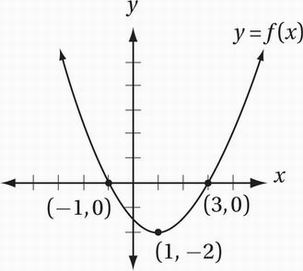# SAT Math Multiple Choice Question 687: Answer and Explanation

### Test Information

Question: 687

12.A portion of the graph of the quadratic function y = f(x) is shown in the xy-plane above. The function g is defined by the equation g(x) = f(x) + b. If the equation g(x) = 0 has exactly one solution, what is the value of b?

• A. -2
• B. -1
• C. 1
• D. 2